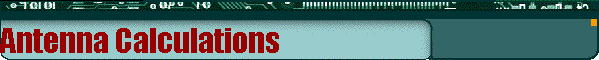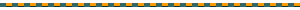[ Up ]

Ground Planes

Halfwave Verticals

5/8th Wave Vertical

Dipoles and Inverted Vees

### Ground Planes

The standard ground plane antenna consists of a vertical radiator which is insulated and centered between 4 horizontal surrounding radials which extend out from the base of the antenna. Each radial is evenly spaced (90 degrees from each other) around the vertical element.

Enter the formula for the antenna calculation (normally 234/f):

divided by Freq. MHZThe vertical length is feet.

The 4 radials are each feet.### Halfwave Verticals

The halfwave vertical antenna consists of a vertical radiator which is fed from the bottom of the antenna. A matching device MUST be added between the antenna and the feedline if you wish to use coaxial. Adding a coil in series with the antenna at the base is one way of doing this.

Enter the formula for the antenna calculation (normally 468/f):

Switch to:

divided by Freq. MHZ

Your halfwave antenna's vertical length isThe cubical quad antenna is a four sided antenna, with each side about a quarter wave length long.  Several factors influence the performance of the antenna, such as :The height above the grounddiameter of the wirenearby structureseffects of other antennas in the areaconductivity of the soil

Enter the formula for the antenna (normally 1005/f):

divided by Freq. MHZEach of the 4 sides are feet.### 5/8th Wave Vertical Antenna

The 5/8 wave antenna consists of a vertical radiator whisc is fed at the bottom of the antenna. A matching device such as a coil in series with the antenna must be used if you wish to feed it with coax.

Enter the formula for the antenna (normally 585/f or 178.308/f for metric):

Switch to:

divided by Freq. MHZYour 5/8 wave antenna's Vertical element is### Dipole and Inverted Vee Antennas

Most people are familiar with the centerfed, halfwave dipoles and inverted vees that are so popular. There is no exact formula for calculating these antennas. The problem is that there are several factors that affect the antenna.

Fortunately there is a starting formula for these types of antennas. For center fed, wire dipole antennas, the formula is 468/freq(mhz).

Enter the formula for the antenna:

divided by Freq. MHZ

Percent smaller for an Inverted Vee.

Your dipole's total length is feet. Each leg of the dipole is feet.Your Inverted Vee's total length is feet. Each leg of the Inverted Vee is feet.